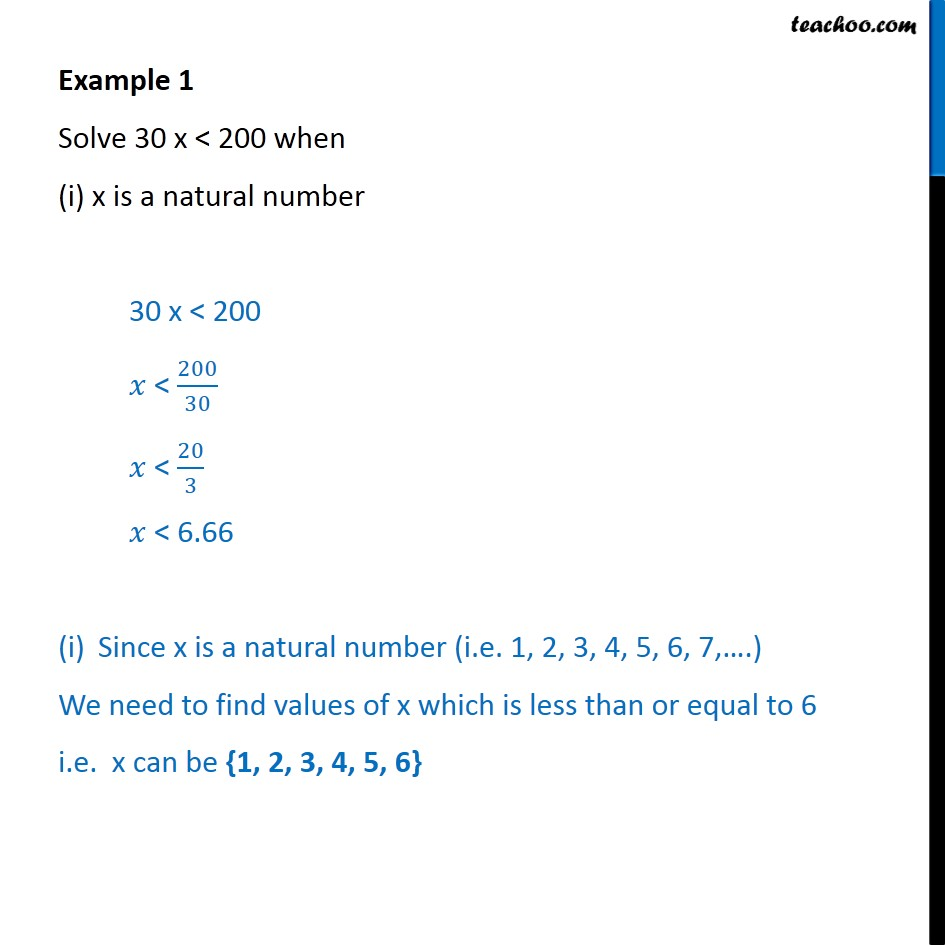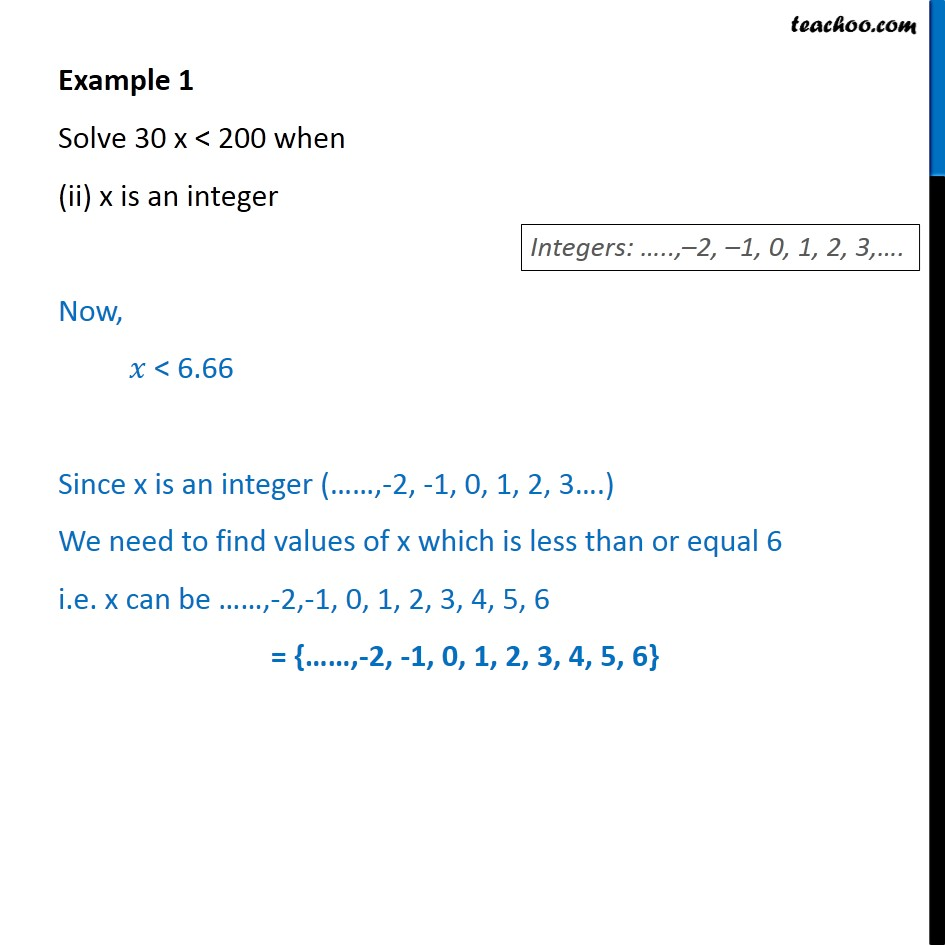1. Chapter 6 Class 11 Linear Inequalities (Term 2)
2. Concept wise
3. Solving inequality (one side)

Transcript

Example 1 Solve 30 x < 200 when (i) x is a natural number 30 x < 200 𝑥 < ﷐200﷮30﷯ 𝑥 < ﷐20﷮3﷯ 𝑥 < 6.66 Since x is a natural number (i.e. 1, 2, 3, 4, 5, 6, 7,….) We need to find values of x which is less than or equal to 6 i.e. x can be {1, 2, 3, 4, 5, 6} Example 1 Solve 30 x < 200 when (ii) x is an integer Now, 𝑥 < 6.66 Since x is an integer (……,-2, -1, 0, 1, 2, 3….) We need to find values of x which is less than or equal 6 i.e. x can be ……,-2,-1, 0, 1, 2, 3, 4, 5, 6 = {……,-2, -1, 0, 1, 2, 3, 4, 5, 6}